# Pi

Pi is one of the greatest numbers of all time, having been known for thousands of years and over that time gaining quite a bit of popularity in the form of celebrations such as Pi Day and others, all from a number that came from the simplest smooth object: A circle. Suppose you have a circle with a width of 1 inch, and then take a measuring tape and measure around the edge. You’ll find that it comes out to 3 inches and a bit, and if you increase the inch to a foot, you might get 3.14 if you look carefully enough. On the more extreme scale, you could go out to a crop circle, measure it, and perhaps get 3.1415926 . Now, suppose you have a perfect circle, and an infinitely precise ruler (for lengths shorter than an atom) , and do the same thing once again. You would get the number 3.141592653589793238462643383… which is expressed as the Greek symbolOne of the first mentions of  pi is in the Bible, where in Kings 7:23-26 it states:

And he [Hiram] made a molten sea, ten cubits from the one rim to the other it was round all about, and…a line of thirty cubits did compass it round about….And it was an hand breadth thick….”
This states that pi=3, a definite approximation, but a terrible one nonetheless. A slightly better approximation was made by Archimedes, when he developed a formula for computing pi by using polygons with large numbers of sides, and getting two approximations for the area of a circle ( pi*r^2) , like this:

Using this method, he drew 2 96-sided polygons and got 3 10/71<pi<3 1/7 , an approximation accurate to 2 decimal places: 3.14… Ptolemy later updated this with 3.141… and this was updated by Tsu Ch’ung Chi to 355/113 , correct to 6 places. Later on, in the 1600s, Gottfried Leibniz/James Gregory found an infinite sum for pi: pi=4*(1-1/3+1/5-1/7…) The proof of this requires calculus, but takes up less than a page. Leibniz’s/Gregory’s formula is rarely used because it takes exponentially many terms to create more digits, which would slow down even the fastest computers. A slightly better formula, but much more amazing, was found by Francois Viete in 1593, using only the number 2!A quite beautiful formula for pi was found by John Wallis, in the form ofNotice how the numerators and the denominators appear to “carry over” to the next fraction!

Shortly thereafter, a much better formula was found by  John Machin in 1706:

Pi/4=4*Arccot(5)-Arccot(239)=4*Arctan(1/5)-Arctan(1/239)

This formula, when expressed in radians, can be computed rapidly using Arccot(x)=1/x-1/(3x^3)+1/(5x^5)-1/(7x^7)… Formulas of this type, arctans of fractions, are now called “Machin-like formulae”.  The simplest of these is Pi/4=Arctan(1), followed by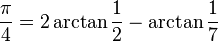andThe arctans with bigger denominators produce more digits per series term, so the efficiency of a Machin-like formula is limited by the arctan with the smallest denominator. For example, the 2002 Pi decimal places record was set by Yasumasa Kanada on a supercomputer using Kikuko Takano’s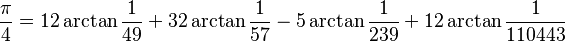and F. C. W. Störmer‘sEven more complicated Machin-like formulae exist, such as Hwang Chien-Lih’s 2002However, in the computer age, the length or the elegance of the formula don’t count: it’s the rate at which the formula converges. Snirvasa Ramanujan, Indian matematician and nemesis of Bill Gosper (“Every time I find an identity, he’s found it before me!”), created a number of formulae for pi,  including the following: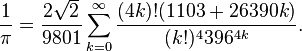wheredenotes f(a)+f(a+1)+f(a+2)…+f(b). Note not only the factorials (n!=1*2*3*4*5…*n) but also the large terms both on the outside and on the inside, especially the factorial to the 4th power and the 396^(4k), which can be shown to mean that the sum converges exponentially rapidly (digits/term), as opposed to exponentially slowly as in the Gregory-Leibniz formula, which makes it one of the fastest algorithms known for computing pi. An even faster algorithm, which has been used to break the pi record many times, is the formula found by the Chudnovsky brothers in 1987: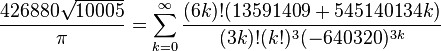This rather monstrous formula gives about  14 digits per term, and was used most recently by Shigeru Kondo and Alexander Yee to calculate 5 trillion digits of pi, billions of times more than enough to estimate the area of your wading pool to the atom. There are even formulae that give an exponential number of digits per iteration, with the drawback that each calculation is exponentially hard. One of these, the Brent-Salamin algorithm, only uses simple arithmetic and would take about 35 iterations to break the record:

First, start with a_0=1,b_0=1/sqrt(2),t_0=1/4,and p_0=1. Then iterate: a_(n+1)=(a_n+b_n)/2, b_(n+1)= sqrt(a_n*b_n), t_(n+1)=t_n-p_n(a_n+a_(n+1))^2, and p_(n+1)=2 p_n. Then when you’ve iterated enough, the estimate for pi is given by (a_n+b_n)^2/(4 t_n).The best of these iterative formulas that I know of is Borwein and Borwein’s, which converges like 9^n (Effectively, it requires about 11 iterations to beat the current record):and then iterateThen the estimate for pi is given by 1/a_n .

A fairly significant formula, found in 1995 by Simon Plouffe, is the Bailey-Borwein-Plouffe formula, which can be used to compute any bit in the hexadecimal representation of pi-without needing to know the previous digits, which can then be used to compute binary bits. In decimal-finding form, it is:This formula was used by PiHex, an ended distributed computing program, to determine that the 1 quadrillionth bit of pi was 0. Yahoo later used the same to find that the 2 quadrillionth bit of pi was also 0.

Of course, the reasons of finding decimal digits of pi are not only to show how great your new supercomputer is, but also to attempt to find a pattern. In base 10, this is probably unlikely, as there are an infinite number of other bases to test, including the non-integer bases(i.e. 7/5ths, sqrt(2),6*e/19…) This makes it practically impossible, and even if base 10 or base 11 or base 16 had a pattern, we might have to look any number of places to find it, as in Carl Sagan’s novel Contact, where (spoiler) after a few trillion digits in base 11, one of the main characters finds a field of 0s and 1s the size of two prime numbers multiplied together. Plotting the 0s and 1s as black and white dots, she plots it on her computer screen to find- a picture of a circle! This is actually possible (though very unlikely) as one of Hardy and Wright’s theorems state that any sequence of digits you can think of can be found in pi. In fact, there’s a website (http://www.dr-mikes-maths.com/pisearch.html) which will search for names in pi expressed in base 26! (end spoiler)

However, there’s a way to express pi in such a way that it doesn’t depend on the base: Continued fractions! Continued fractions are “infinite fractions” which are in the form of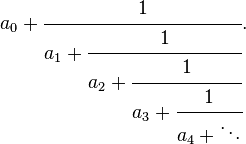and are usually expressed as [a0,a1,a2,a3,a4,a5,…] or as [a0;a1,a2,a3,a4,a5,…] with all an positive integers. Many numbers, such as integers and fractions, have rational continued fractions: For example, 1=, and 355/113=[3,7,15,1]. Of course, if 355/113 were expressed in decimal, you’d have to use an infinite number of digits to get the actual fraction. A significant advantage that continued fractions have over decimal notation is that often irrational numbers can be expressed as repeating continued fractions. For example,

sqrt(2)=1.4142135623730950488016887242097… but in continued fraction notation

sqrt(2)=[1;2,2,2,2,2,2,2,2,2,2,2,2,2,2,2,2,2,2,2,2,2,2,…]

Much simpler. In fact, you can go to your friends, claim you know more digits of the square root of 2 than them, and you can simply expand the continued fraction out to beat them no matter how many decimal digits they know! Possibly the most elegant of these repeating continued fractions is the one for the Golden Ratio, (1+sqrt(5))/2:Also, sometimes transcendental numbers can be expressed as simple continued fractions. For example, Euler’s Number, e, is equal to lim(n->infinity) (1+1/n)^n and is often used in exponentiation and calculus. In continued fraction form, it is equal to [2,1,2,1,1,4,1,1,6,1,1,8,1,1,10,1,1…]! Decimal is not as elegant, e being about 2.71828182845904523536…

However, despite any hope, Pi is not as pretty in continued fraction form, though it is invariant of base: [3,7,15,1,292 (ack!),1,1,12,1,3,1,14,2,1,1,2…] There have been only a few attempts for the continued fraction of pi; Tsu Ch’ung Chi’s 355/113=[3,7,15,1] was the first nontrivial one, and Euclid’s algorithm can be used for computing the continued fraction of pi, though his GCD algorithm just throws the terms away. The first major record that I know of was made by Bill Gosper on August 19,1977 when he computed 204103 terms using his own algorithm in Macsyma, an early computer algebra system. Later, he beat his own record  in 1985 with a whopping 17001303 terms, again using his algorithm. Later, in 1999 Hans Havermann beat Gosper’s record by using Mathematica to compute 20,000,000 terms. He later beat this in March 2002 to make 180,000,000 terms, the previous record.

Now might be a good time to tell why I haven’t been blogging recently.

Over the past few months, I have been working on a C# program, PiCF (not released yet, current alpha source code here) which can calculate the continued fraction of any number, not just pi, using Gosper’s algorithm. On October 17th, I calculated approximately 458,000,000 terms of pi in about 3 hours on a 64-bit machine running Windows on a Core 2 Duo @ 3.00 Ghz. This was later verified using Mathematica (taking up much more memory than the calculation did!). The program was coded in C#, has a command-line interface (with menus!), and uses Emil Stefanov’s wrapper of GNU MP for the BigInteger multiplications. The maximum term is still 878783625, originally found by Bill Gosper during the 17M calculation.  Other stats: The minimum term is one (naturally),  the terms take a 1.4 GB file (download here if you dare) and I was very nervous.

Pi has, over the years, gained a huge following: March 14th is Pi Day (in decimal) on which MIT ships their student acceptments/declines and on which people make pie, 1:59:26 of that day is Pi Second; May 22nd is Pi Approximation Day, and Kate Bush even wrote a song about pi. Many jokes about pi have surfaced on the Internet, including that:This may be because over the thousands of years, pi has become so far removed from its original purpose: measuring circles. To start out, almost every round object has a volume and surface area that involves pi. The volume of a cone is one-third r^2 *h*pi, where r is the radius of the base and h is the height. The volume of a torus is (pi^2)/4*(b+a)(b-a)^2 where a is the inner radius and b is the total radius.

What about the volume of a crescent? Well, that’s quite a different story…From Murderous Maths: The Perfect Sausage by Kjartan Poskitt

## 10 thoughts on “Pi”

1.Bram Cohen says:

Are the details of Gosper’s algorithm online somewhere?

The formula for directly finding the Nth digit of pi you give is for hexadecimal digits, not decimal digits. The paper giving the formula says that the authors don’t know of a decimal formula, but believe that such a beast exists.

Do all algebraic numbers have repeating continued fraction representations?

1.nbickford says:

Ah, I must’ve forgotten that. I have some details on the algorithm on the official announcement page, but I’ll reprint the email he sent to me about it:

Not so hard. Input: num(erator), denom(inator), prec(ision) (in bits).
Output: the 2×2 matrix [a,c;b,d] where a/b is the whole cf and c/d is same w/o
last term–i.e. like Macsyma’s CFEXPAND. Both a/b and c/d will be “best”
approximations to num/den.
Side effect: output the cf terms (in fractal bursts) to a stream going into a
normalizer–an identity function with a small, built-in delay to smooth out
nonpositive terms.

Method: Shorten (right shift) num and den so that the smaller has prec bits, maybe plus a few.
If prec = tiny, just run Euclid’s algorithm on num/denom, streaming the terms and returning
the required matrix (= [t1,1;1,0] . [t2.1;1,0]…, where t1,t2,… are the Euclid terms.)
Otherwise: recurse on the first prec/2 bits of num and denom, and prec/2,
getting [a,c;b,d]. Then recurse on c*den-d*num and b*num-a*den (which should be half the
prec of num and den) and prec/2, getting [a’,c’;b’,d’]. Return [a,c;b,d].[a’,c’;b’,d’] .
Debug a toy one; then scale it up.
–Bill
There are some things that he doesn’t mention, such as that the function that you have to use for the right shortening on num and denom is Min((BitLength(Max(Abs(denominator),Abs(numerator))) – prec),BitLength(denominator)-1); to quietly sweep under the carpet cases when the denom is 0 (as happened once during testing around the 450,000th term). Also, you need to halt Euclid’s algorithm when it is half depleted (otherwise it spits out terms, then a 0, and then the negative of some of those terms,the CF equivalent of a take-back) as well as having the first recursion go with floor(prec/2) and the second use floor(prec/2)+1. In case it helps, I also have the current source code up on my website (http://neilbickford.com/source.txt), but it’s not very well documented.

Not all algebraic numbers have a repeating continued fraction representation; The cube root of 5 doesn’t repeat within the first 1,000,000, and the square root of 2 plus the square root of 3 doesn’t repeat within the first 1,000,000. All continued fractions that are arithmetic functions of continued fractions (e.g. addition, subtraction, multiplication, division) seem to have repeating continued fractions of the form [a0;,,…] There’s a good paper on continued fractions of algebraic numbers at http://citeseerx.ist.psu.edu/viewdoc/download?doi=10.1.1.135.107&rep=rep1&type=pdf and Gosper did an item in HAKMEM about continued fraction arithmetic: http://www.inwap.com/pdp10/hbaker/hakmem/cf.html#item101a
–Neil

2.Schuyler Hartje says:

i do like pie. 😀

3.stan wagon says:

You wrote: This is actually possible (though very unlikely) as one of Hardy and Wright’s theorems state that any sequence of digits you can think of can be found in pi

This does not sound right to me. I thought it was not proved that all digits occur infinitely often. So, for example, pi could, after some point, involve only 0s and 1s (in base 10). If the cited theorem was correct, of course one would have all digits appearing infinitely often.

Stan Wagon, Macalester College

4.1111 says:

What do you think about tau?

I think it’s a better number than pie.

See vi hart’s youtube videos for reasons why 🙂

5.mrbigglesw0rthPaul says:

I shall subscribe to your newsletter. Wolframalpha, Mathmatica and others need to be a part of our public school system.

6.Anonymous 1763 says:

stan wagon :
You wrote: This is actually possible (though very unlikely) as one of Hardy and Wright’s theorems state that any sequence of digits you can think of can be found in pi
This does not sound right to me. I thought it was not proved that all digits occur infinitely often. So, for example, pi could, after some point, involve only 0s and 1s (in base 10). If the cited theorem was correct, of course one would have all digits appearing infinitely often.

I do know that it has not been proven whether or not pi is normal; however, I think (A) “is normal” is not quite equivalent to (B) “any finite digit-sequence appears at least once”. Of course either A or B would imply (C) “all digits appear infinitely often”, so if you’re right about the unprovenness of C, then at least one of Neil, Hardy, and Wright must be wrong about the provenness of B.

7.stan wagon says:

No citation was given for the Hardy and Wright result, so I cannot tell. I have my Hardy and Wright “Intro to Th. of Numbers” in front of me. One wonders if Neil is referring to something in that book. Again, my understanding is that, as far as we know, pi could, in base ten, be something like 3.14159…..1011011110101011101011111110…..

Stan Wagon